ChemTalk

# Reaction Order – First & Second Order## Core Concepts

In this article, we learn all about order of reaction, including its importance, its effect on the rate constant and rate law, and how to calculate it using kinetic data. You will learn what a first order reaction is, and a second order reaction, along with other reaction orders.

## What is Order of Reaction?

In chemistry, reactions can occur fast, slow, or anywhere in between. Chemists tend to characterize the kinetics of a reaction through a rate law. This rate law tends to depend on the concentrations of one or more reactants. The reaction rate equals the rate of product formation or reactant consumption, adjusted by stoichiometric coefficients.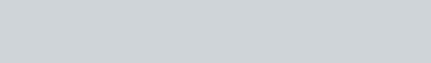In rate laws, the reactant concentrations are raised to some power (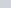and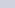). Chemists call these values the reaction order, with respect to each reactant.

The sum of the two order reactant reaction orders yields the overall reaction order. The overall order of reaction has a lot of importance to chemists since it determines the reaction’s rate law and integrated rate law.

Notice that these reaction orders have no dependence on the stoichiometry of the reaction. As we explore in the next section, reaction order instead depends on the mechanism of the reaction. For instance, we can imagine that the above reaction is first-order with respect to reactant A and second-order with respect to reactant B, yielding a third-order reaction.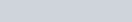We can also imagine reactions that have a zeroth-order reactant. In such cases, the overall rate completely depends on the concentration of the other reactant or no reactant.In truth, reactions with an overall order of zero tend to be rare. However, chemists have found some examples, which tend to involve a catalyst or enzyme in much lower concentrations than the reactants. In these cases, the steady state approximation often applies. For instance, for all intents and purposes, the enzymatic degradation of ethanol to acetaldehyde has zeroth-order kinetics.

## Zeroth Order Reactions

In chemistry, a zero-order reaction refers to a specific type of chemical reaction that is independent of the concentrations of any reactant. Let’s say we have a reaction where the starting rate of the reaction is “k”. A zero-order reaction means that the rate at which the the products transform into the reactants will always be k, no matter what the concentrations of these products and reactants are, or how far the reaction has progressed.

In a zero-order reaction, the rate of the reaction is always some constant. As the concentration of the reactant decreases, the rate remains the same. This means that the rate of the reaction is constant throughout the process.

Mathematically, we represent a zero-order reaction using an equation like this: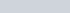Here,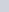is a constant that represents the reaction rate constant. The equation shows that the rate of the reaction is always, and no other variables can impact this rate.

So, in summary, a zero-order reaction is a type of chemical reaction where the rate of the reaction doesn’t depend on the concentration of any reactant. As the concentration of that reactant decreases, the reaction slows down.

## First Order Reactions

In chemistry, a first-order reaction refers to a specific type of chemical reaction that depends on the concentration of only one reactant. Let’s say we have a reaction where a substance, let’s call it “A,” is transforming into another substance, let’s call it “B.” A first-order reaction means that the rate at which A transforms into B is directly proportional to the concentration of A.

Imagine you have a bottle filled with a colored liquid, and you want to measure how fast the liquid changes color over time. Initially, the bottle is full, so there are lots of molecules of the colored substance in the liquid. As time passes, the number of molecules decreases because they’re being converted into another substance or disappearing from the reaction.

In a first-order reaction, the rate of the reaction is determined by the concentration of A. As the concentration of A decreases, the reaction slows down. This means that the rate of the reaction is not constant throughout the process but instead changes as the concentration changes.

To visualize this, think of the bottle with the colored liquid. At the beginning, when the concentration of A is high, the reaction occurs quickly because there are many A molecules available to react. However, as time goes on and the concentration of A decreases, the reaction becomes slower because there are fewer A molecules left to react.

Mathematically, we represent a first-order reaction using an equation like this: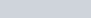Here,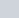represents the concentration of substance A, andis a constant that represents the reaction rate constant. The equation shows that the rate of the reaction is directly proportional to the concentration of A.

So, in summary, a first-order reaction is a type of chemical reaction where the rate of the reaction depends on the concentration of only one reactant. As the concentration of that reactant decreases, the reaction slows down.

## Second Order Reactions

In chemistry, a second-order reaction refers to a specific type of chemical reaction that depends on the concentrations of two reactants. Let’s say we have a reaction where two substances, A and B, come together to form a new substance, C. In a second-order reaction, the rate at which A and B react is determined by the product of their concentrations.

To understand this concept, let’s imagine you have two containers, one filled with red marbles and the other with blue marbles. The marbles represent the molecules of substances A and B. Initially, both containers are full, so there are plenty of red and blue marbles. As the reaction proceeds, the marbles from both containers come together and form a new container with purple marbles representing substance C.

In a second-order reaction, the rate of the reaction depends on the concentrations of both A and B. As the concentrations of A and B decrease, the reaction slows down. This means that the rate of the reaction is not solely dependent on the concentration of one reactant but rather on the product of their concentrations.

Mathematically, we represent a second-order reaction using an equation like this: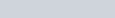Here,and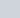represent the concentrations of substances A and B, respectively, andis a constant that represents the reaction rate constant. The equation shows that the rate of the reaction is directly proportional to the product of the concentrations of A and B.

To visualize this, imagine that you have more red marbles than blue marbles in the containers. At the beginning, when both concentrations are high, the reaction occurs quickly because there are many A and B molecules available to react. However, as time goes on and the concentrations of A and B decrease, the reaction slows down because there are fewer A and B molecules left to react.

So, in summary, a second-order reaction is a type of chemical reaction where the rate of the reaction depends on the concentrations of two reactants. As the concentrations of both reactants decrease, the reaction slows down.

## Reaction Order and Rate Constants

As you may have noticed, each rate law involves some constant “”. Chemists call this value the rate constant, which serves to adjust reactant concentration values to equal the reaction rate. Most importantly, the rate constant adjusts the rate law’s units in order to yield a rate in molar per second (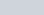).

To fulfill this, the units of the rate constant can vary depending on the overall order of reaction. For zeroth-order reactions, the rate constant has units of molar per second (). In first-order reactions, the rate constant has units of “per second” (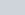). For second-order reactions, the rate constant has units of “per molar per second” (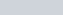). For each successive reaction order, the power of the molar decreases by one.

Zeroth-Order: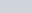Units: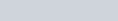First-Order: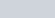Units: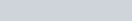Second-Order: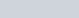Units: (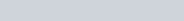Third-Order: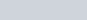Units: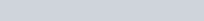## How to find Order of Reaction

The methods for finding reaction order, overall and for given reactants, are the same for finding the rate law. This is because you need to know reaction order to write a rate law.

#### Rate Determining Step Method

As mentioned before, the order of reaction depends on the specific mechanism of the reaction, rather than the stoichiometry. Many reaction mechanisms involve multiple elementary steps. For instance, the SN1 reaction from organic chemistry involves two steps: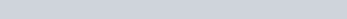• Step 1: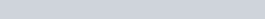• Step 2: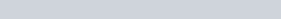Importantly, Step 1 occurs at a much slower rate than Step 2. As a result, Step 1 serves as the rate-determining step, which means that it equals the overall rate of reaction. Therefore, our rate-determining step yields our rate law, depending on the stoichiometry of the reactants in that step: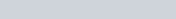To be clear, only the stoichiometry of the rate-determining step influences the rate law not the stoichiometry of the overall reaction.

Since only one molecule of reactant participates in the rate-determining step, the SN1 must therefore be first-order overall. Further, since tert-butyl bromide ((H3C)3CBr) has a stoichiometric coefficient of 1, the reaction must be first-order with respect to tert-butyl bromide. Hydroxide (OH) does not participate in the rate-determining step, thus making the reaction zeroth-order with respect to hydroxide.

#### Empirical Data Method

However, if you don’t know the mechanism and rate-determining step of a reaction, you can instead determine reaction orders through empirical data. Specifically, you need to perform multiple trials of a reaction, adjusting the concentration of reactants. The concentrations of reactants thus affect the reaction rate depending on their reaction orders, which you can measure using spectrophotometry. The Iodine Clock Reaction provides a good example of a reaction whose kinetics can be determined through this method.

The Iodine Clock Reaction proceeds by the following reaction: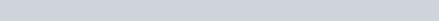Triiodide forms a complex that absorbs blue light (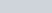), which means that a spectrophotometer can therefore measure its rate of formation. To find the reaction order, you run this reaction five times, varying the initial concentrations of each reactant:

By comparing specific trials, we find that disulfur trioxide and aqueous protons do not affect reaction rate, while iodide and hydrogen peroxide have a one-to-one effect. This therefore means that the reaction is first-order with respect to iodide and hydrogen peroxide and zeroth-order for the others. Overall, the reaction is second-order.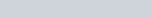If you’d like to see an in-depth analysis of kinetic data to yield reaction order and rate law, check out this article.

## Rate Law Practice Problems

Problem 1

For the reaction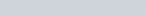, you observe the following data:

What is the rate law for this reaction?

Problem 2

You have the following reaction and rate law:

(1)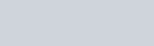What reactants would you expect to have in the rate-determining step?

Problem 3

Let [A] be the concentration of a reactant in a chemical reaction. In an experiment, when you measure the concentration of [A] over time, you get the following group (see below). What is the order of the reaction?

## Rate Law Practice Problem Solutions

1: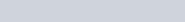2: A only

3: 0th order, because the rate (this is the slope, the change in the concentration of [A] over time) is constant throughout the reaction!